Zen Pharaohs of Ultimate Love mathematics, music, science, wood....what else could you ask?Next: Bidiagonal Representation of TIB Up: Triangular Input Balanced Realizations Previous: Meixner Functions and TIB

## Gauss-Meixner Summation

The impulse response of a TIB realization with a single eigenvalue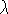is given by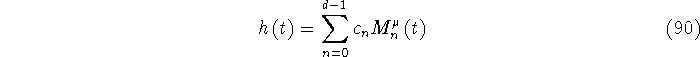where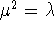and, as usual, c is the vector of coefficients. In order to compute c we exploit Gaussian quadrature (in this case summation) properties of the orthogonal polynomials. The normalized three-term recurrence for the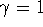Meixner polynomials results in the Jacobi matrix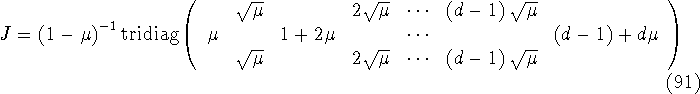and the associated Gauss-Meixner summation formula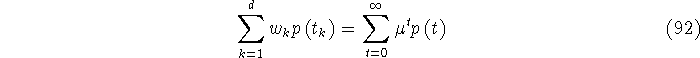where p is any polynomial of degree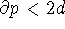. The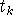are the eigenvalues of J and the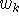are proportional to the squares of the first components of the corresponding orthonormal eigenvectors. We fix theby choosing the proportionality constant so thatfor consistency, (i.e. consider p=1).

We put this in terms of a summation formula for the Meixner functions:This allows us to compute Meixner coefficients, that is for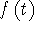of the form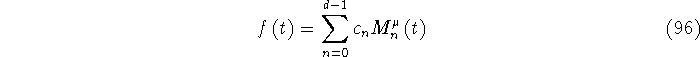by the formulaNow we can compute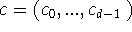recursively without explicitly computing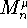as follows.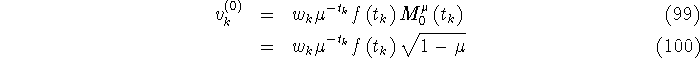then using the recursion for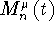: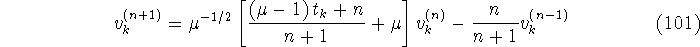and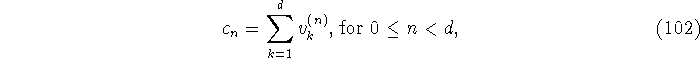where we put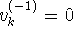. A check that this has worked is that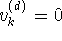for k=1,...,d.

Gauss-Meixner summation works very well when we can bound the order d of the model in advance, and it is one attractive way of computing the TIB filter realization of the maximally flat impulse response.

Fri Jun 27 03:10:38 EDT 1997